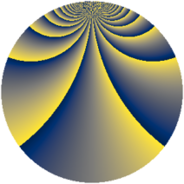# Properties

 Label 1792.2.bcLevel $1792$ Weight $2$ Character orbit 1792.bc Rep. character $\chi_{1792}(113,\cdot)$ Character field $\Q(\zeta_{16})$ Dimension $384$ Sturm bound $512$

# Learn more

## Defining parameters

 Level: $$N$$ $$=$$ $$1792 = 2^{8} \cdot 7$$ Weight: $$k$$ $$=$$ $$2$$ Character orbit: $$[\chi]$$ $$=$$ 1792.bc (of order $$16$$ and degree $$8$$) Character conductor: $$\operatorname{cond}(\chi)$$ $$=$$ $$64$$ Character field: $$\Q(\zeta_{16})$$ Sturm bound: $$512$$

## Dimensions

The following table gives the dimensions of various subspaces of $$M_{2}(1792, [\chi])$$.

Total New Old
Modular forms 2112 384 1728
Cusp forms 1984 384 1600
Eisenstein series 128 0 128

## Trace form

 $$384q + O(q^{10})$$ $$384q + 64q^{51} + 128q^{55} + 80q^{63} + 80q^{67} + 128q^{75} + 64q^{79} + O(q^{100})$$

## Decomposition of $$S_{2}^{\mathrm{new}}(1792, [\chi])$$ into newform subspaces

The newforms in this space have not yet been added to the LMFDB.

## Decomposition of $$S_{2}^{\mathrm{old}}(1792, [\chi])$$ into lower level spaces

$$S_{2}^{\mathrm{old}}(1792, [\chi]) \cong$$ $$S_{2}^{\mathrm{new}}(64, [\chi])$$$$^{\oplus 6}$$$$\oplus$$$$S_{2}^{\mathrm{new}}(256, [\chi])$$$$^{\oplus 2}$$$$\oplus$$$$S_{2}^{\mathrm{new}}(448, [\chi])$$$$^{\oplus 3}$$